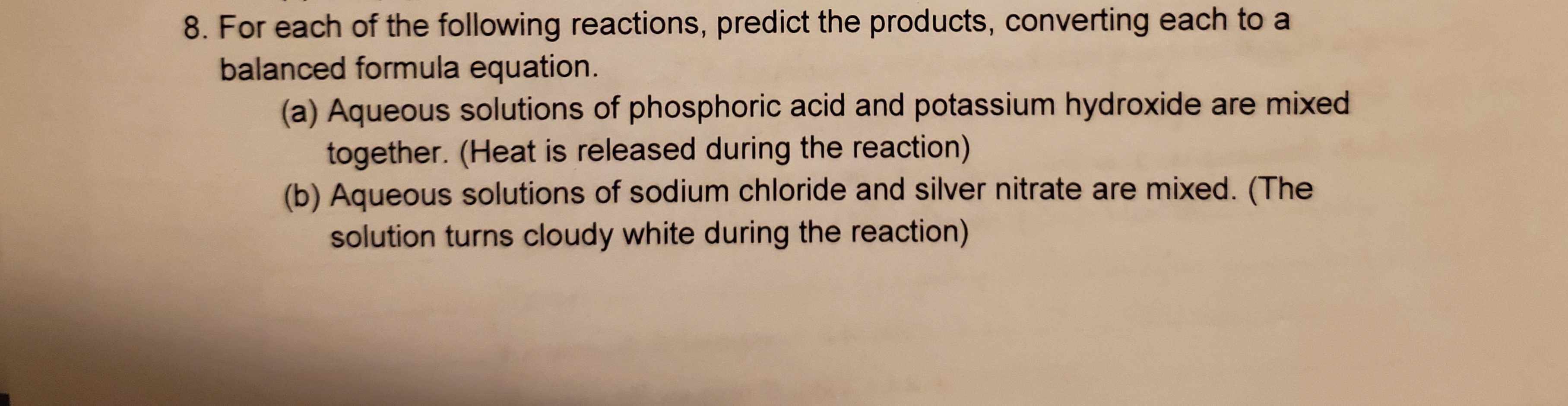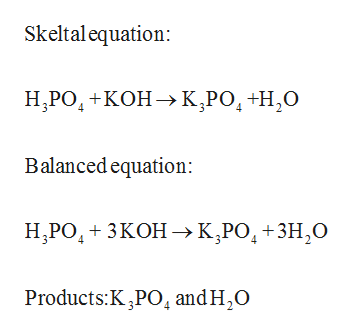# 8. For each of the following reactions, predict the products, converting each to abalanced formula equation.(a) Aqueous sol utions of phosphoric acid and potassium hydroxide are mixedtogether. (Heat is released during the reaction)(b) Aqueous solutions of sodium chloride and silver nitrate are mixed. (Thesolution turns cloudy white during the reaction)

Question
22 viewshelp_outlineImage Transcriptionclose8. For each of the following reactions, predict the products, converting each to a balanced formula equation. (a) Aqueous sol utions of phosphoric acid and potassium hydroxide are mixed together. (Heat is released during the reaction) (b) Aqueous solutions of sodium chloride and silver nitrate are mixed. (The solution turns cloudy white during the reaction) fullscreen
check_circle

Step 1

Balanced chemical equation of a reaction is written according to law of conservation of mass.

General Steps in writing a balanced equation,

• Skeleton equation has to be written.
• Count the atoms of the reactants and products.
• Change the coefficient to make the number of atoms of each element equal on both sides of the equation.
Step 2

Given that aqueous solutions of phosphoric acid a...help_outlineImage TranscriptioncloseSkeltalequation: Н,РО, +КОН -> К,РО, +Н,О Balanced equation: Н. РО, + 3КОН > К,РО, +3Н,0 -зн,о Products:K ,PO4 and H2O fullscreen

### Want to see the full answer?

See Solution

#### Want to see this answer and more?

Solutions are written by subject experts who are available 24/7. Questions are typically answered within 1 hour.*

See Solution
*Response times may vary by subject and question.
Tagged in

### General Chemistry